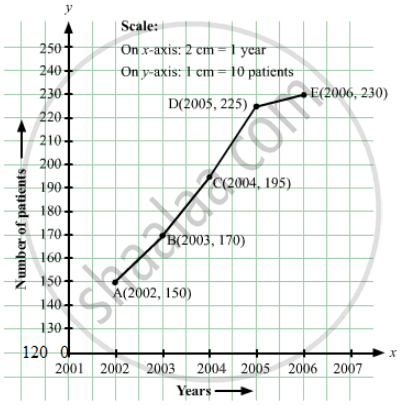Advertisement Remove all ads

# The Following Table Shows the Number of Patients Discharged from a Hospital with Hiv Diagnosis in Different Years: - Mathematics

Answer in Brief

The following table shows the number of patients discharged from a hospital with HIV diagnosis in different years:

 Years: 2002 2003 2004 2005 2006 Number of patients: 150 170 195 225 230

Represent this information by a graph.

Advertisement Remove all ads

#### Solution

Here, years is an independent variable and the number of patients is a dependent variable. So, we take years on the x-axis and the number of patients on the y-axis.
Let us choose the following scale:
On x-axis: 2 cm = 1 year
On y-axis: 1 cm = 10 patients
Also, let us assume that on the x-axis, origin (O) represents 2001 and on the y-axis, origin (O) represents 120, i.e. O (2001, 120).
Now, let us plot (2002, 150), (2003, 170), (2004, 195), (2005, 225), (2006, 230). These points are joined to get the graph representing the given information as shown in the figure below.Is there an error in this question or solution?
Advertisement Remove all ads

#### APPEARS IN

RD Sharma Class 8 Maths
Chapter 27 Introduction to Graphs
Exercise 27.2 | Q 1 | Page 15
Advertisement Remove all ads
Advertisement Remove all ads
Share
Notifications

View all notifications

Forgot password?
Course